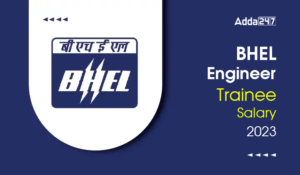Engineering Jobs   »   Mechincal Engineering quizs   »   UPPSC Lecturer Recruitment 2021, UPPSC Lecturer...

# UPPSC Lec’21 ME: Daily Practices Quiz. 03-Dec-2021

Quiz: Mechanical Engineering
Exam: UPPSC AE/Lecturer
Topic: Engg. Mechanics

Each question carries 3 marks
Negative marking: 1 mark
Time: 8 Minutes

Q1. Two balls are dropped from the top of a tower after an interval of 1 second. If the acceleration due to gravity is 10m/s^2 then the separation distance 3 second after the release of the first ball will be
(a) 5 m
(b) 15 m
(c) 25 m
(d) 30 m

Q2. A car travels from one place to another with the uniform speed of 40 km/hr for half distance and with the uniform speed of 60 km/hr for remaining half distance. The average speed of car is
(a) 40 km/hr
(b) 45 km/hr
(c) 48 km/hr
(d) 50 km/hr

Q3. During head-to-head collision of two bodies, the relative velocity of the two bodies after impact is _____ the relative velocity of the two bodies before impact if the impact is elastic impact.
(a) equal to
(b) greater than
(c) less than
(d) equal and opposite to

Q4. A body of mass 10 kg is lifted up through a height of 2 m. How much work is done? (Take g = 9.81 m/s^2)
(a) 49 J
(b) 98 J
(c) 392 J
(d) 196 J

Q5. The unit of energy in S.I unit is
(a) Dyne
(b) Watt
(c) Newton
(d) Joule

Q6. The wheels of a moving car possess
(a) kinetic energy of translation only
(b) kinetic energy of rotation only
(c) kinetic energy of translation and rotation both
(d) strain energy

Solutions

S1. Ans. (c)
Sol. When the first ball is dropped then it travels for 3 seconds under gravity with acceleration g and it has initial velocity u = 0
s=ut+1/2 gt^2
s=0+1/2×10×9=45 m
After 1 second, second ball is dropped so it travels for only 2 seconds then,
s=ut+1/2 gt^2
s=0+1/2×10×4=20
So, distance between two=45-20=25m

S2. Ans. (c)
Sol. We know that,
Average speed = (Total distance)/(Total time )
Let the total distance be d
Average speed =(d/2+d/2)/((d/2)/40+(d/2)/60)=48 km/hr

S3. Ans. (d)
Sol. During elastic impact of two bodies, the relative velocity of the two bodies after impact is equal and opposite to the relative velocity of the two bodies before impact.

S4. Ans. (d)
Sol. Work done to lift a mass of 10 kg up-to 2 m
W = Force × distance
W=m×g×4=10×9.81×2=196.2 J

S5. Ans. (d)
Sol. The unit of energy in S.I unit is Joule.

S6. Ans. (c)
Sol. The wheels of a moving car possess both kinetic energy of translation and rotation.

Sharing is caring!

•GATE 2023 Admit Card Out, Direct Link fo...
•BHEL Engineer Trainee Salary 2023, Perks...
•BHEL Engineer Trainee Cutoff Marks 2023,...
•UPSC ESE Admit Card 2023, Download Preli...
•Last Week Preparation Strategy for GATE ...
•BARC OCES Syllabus 2023 Checkout Latest ...
•SAMEER Recruitment 2023 Out For 35 Posts...